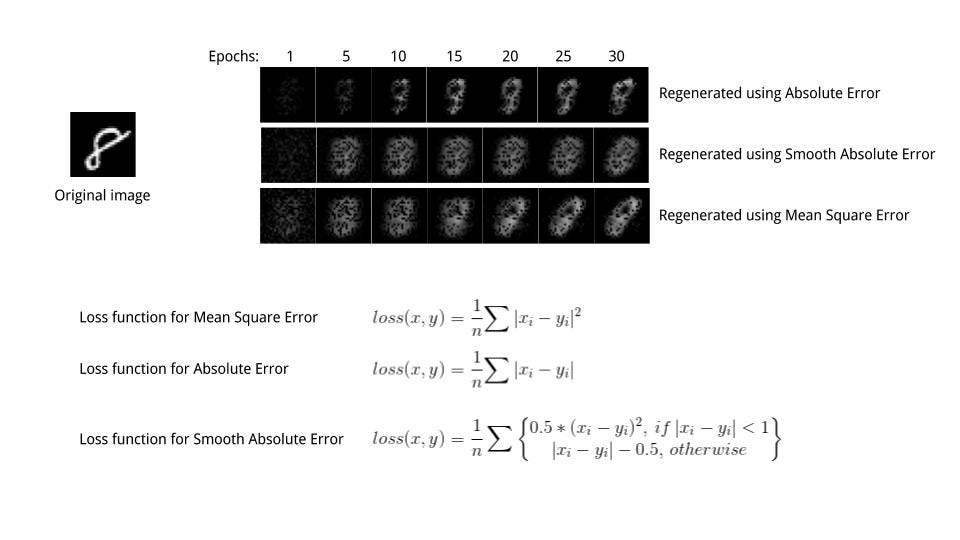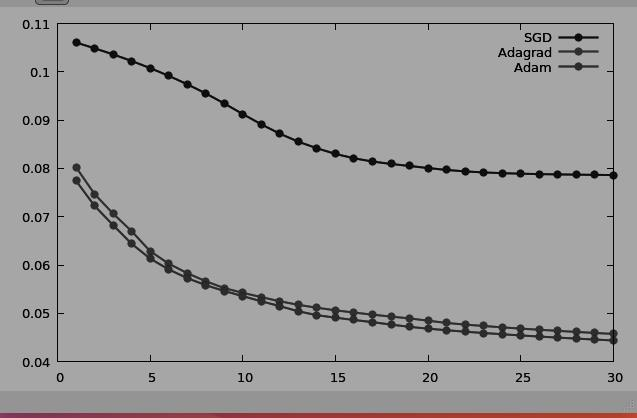## Visualizing Loss Functions

Saturday, Dec 1, 2018| Tags: Deep Learning, Visualization, Loss Functions, Lua# Visualising different loss and optimisation functions using Autoencoder.

The aim of the project was to reconstruct images with the help of Autoencoders to visualise the difference in output when different loss or optimisation functions are used. A very simple dataset, MNIST dataset was used for this purpose.

While training a neural network, gradient is calculated according to a given loss function. I compared the results of three regression criterion functions.

1. Absolute criterion
2. Mean Square Error criterion
3. Smooth L1 criterion

While the Absolute error just calculated the mean absolute value between of the pixel-wise difference, Mean Square error uses mean squared error. Thus it is more sensitive to outliers and pushes pixel value towards 1 (in our case, white as can be seen in image after first epoch itself)

Smooth L1 error can be thought of as a smooth version of the Absolute error. It uses a squared term if the squared element-wise error falls below 1 and L1 distance otherwise. It is less sensitive to outliers than the Mean Squared Error and in some cases prevents exploding gradients.

Optimization algorithms helps us to minimize the Loss functions by updating Weights and Biases and reach to the global minima. Some algorithms give faster and slightly better results based on the dataset. We used three first order optimisation functions: